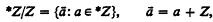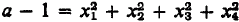Home

# Addition in nonstandard models of arithmetic

## Extract

In  Kemeny made the following conjecture: Suppose *Z is a nonstandard model of the ring of integers Z. Letand let F be the subgroup of those cosets ā which contain an element of infinite height in *Z. Kemeny then asked if the ring R = {a: ā ∈ F} is also a nonstandard model of Z. If so then Goldbach's conjecture is false because Kemeny also shows in  that Goldbach's conjecture fails in R.

The papers  and  by Gandy and Mendelson show that R is not a nonstandard model of Z but we give here a simpler proof based on Mendelson's paper. Suppose R is a nonstandard model of Z. Then each positive number in R is a sum of four squares. Choose a in R so that a is a positive element of R of infinite height in *Z. Then since a is infinite in *Z, a − 1 is positive. Thus, xiR for i = 1, …, 4. Now each xi must be of the form ai + ni, where ai has infinite height in *Z and ni, ∈ Z.

Hide All

# Addition in nonstandard models of arithmetic

## Metrics

### Full text viewsFull text views reflects the number of PDF downloads, PDFs sent to Google Drive, Dropbox and Kindle and HTML full text views.

Total number of HTML views: 0
Total number of PDF views: 0 *Loading metrics...

### Abstract viewsAbstract views reflect the number of visits to the article landing page.

Total abstract views: 0 *Loading metrics...

* Views captured on Cambridge Core between <date>. This data will be updated every 24 hours.

Usage data cannot currently be displayed.